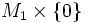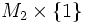# Cobordism of submanifolds

(diff) ← Older revision | Latest revision (diff) | Newer revision → (diff)
Suppose$M_1$ and$M_2$ are submanifolds of a differential manifold$P$. A cobordism of submanifolds from$M_1$ to$M_2$ is a submanifold with boundary$(N, \partial N)$ in$P \times I$, that provides a cobordism between$M_1 \times \{ 0 \}$ and$M_2\times \{ 1 \}$.